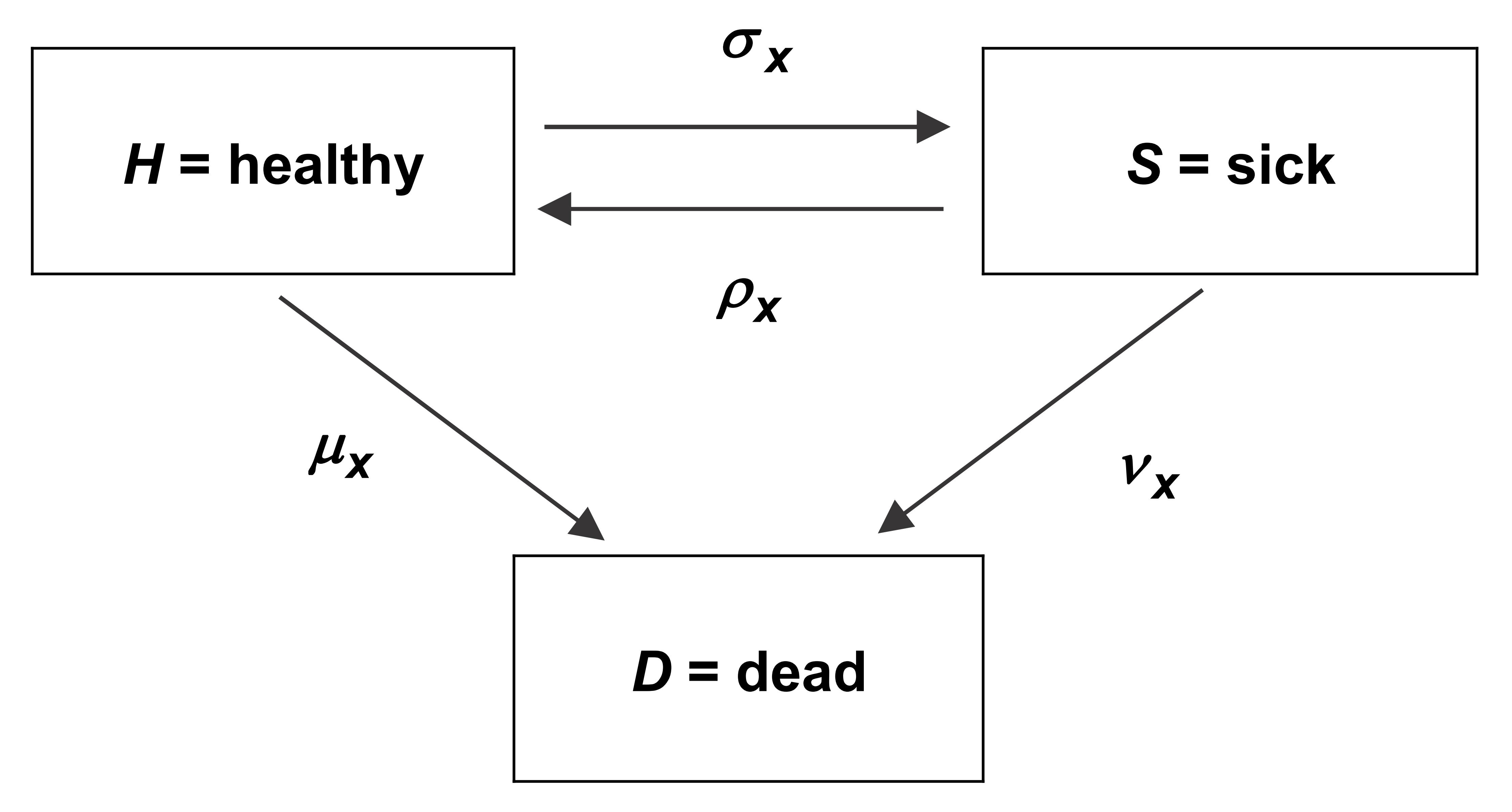IFoA 英国精算师考试 CT4 精算模型的学习大纲，备考必看。各章的主干知识点和考点总结。

CT4分为两部分，1-6章为随机过程，7-13章为生存模型。

## 随机过程部分

### Chapter 4 The two-state Markov model

$\px{t}{x}=exp(-\int^t_0 \mu_{x+s}ds)$

### Chapter 5 Time-homogeneous Markov jump processes

HSD model（health-sickness-death model）的图像非常重要，后续的很多例子都基于此。$p_{01}(x, x+t)=\frac{\mu_{01}}{\mu_{01}+\mu_{02}}\left[1-e^{-\left(\mu_{01}+\mu_{02}\right) t}\right]$

$p_{02}(x, x+t)=\frac{\mu_{02}}{\mu_{01}+\mu_{02}}\left[1-e^{-\left(\mu_{01}+\mu_{02}\right) t}\right]$

## 生存模型部分

### Chapter 11 Exposed to risk

• aged $$x$$ last birthday：上一个生日的年龄为 $$x$$

• aged $$x$$ next birthday：下一个生日的年龄为 $$x$$

• aged $$x$$ nearest birthday：最近的一个生日的年龄为 $$x$$## 结语

CT4 最重要的概念是死亡力。CT4中的每一章都是和死亡力相关的，无论是直接或者间接。Force of mortality，transaction rates，比例风险模型中的hazard，这些都是指死亡力。

You have three methods of graduation, but only one way to pass the exam——work hard.